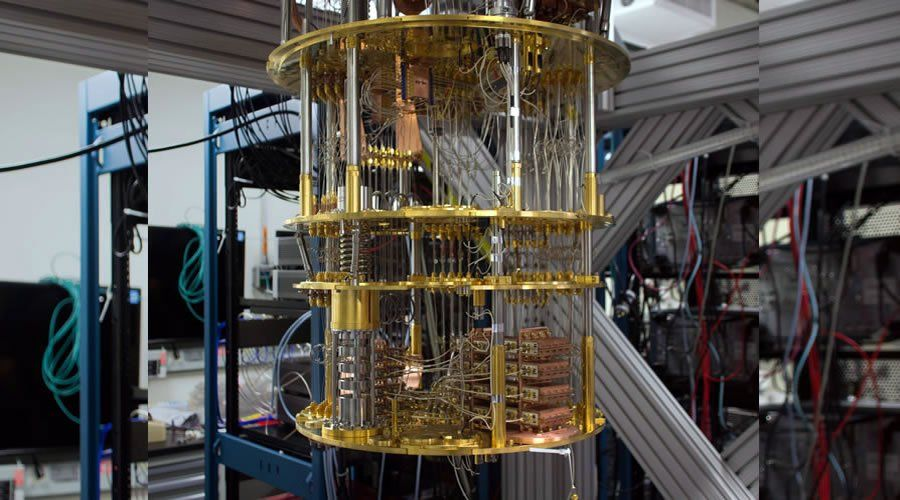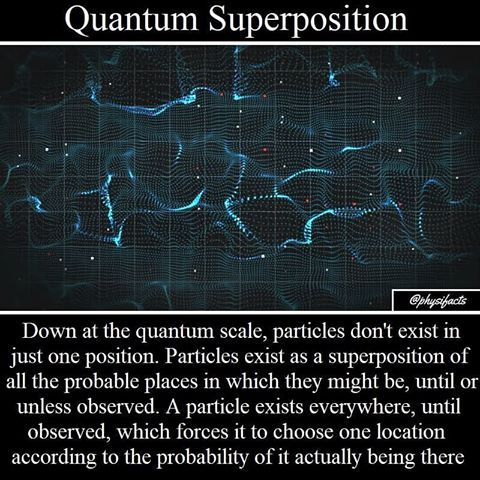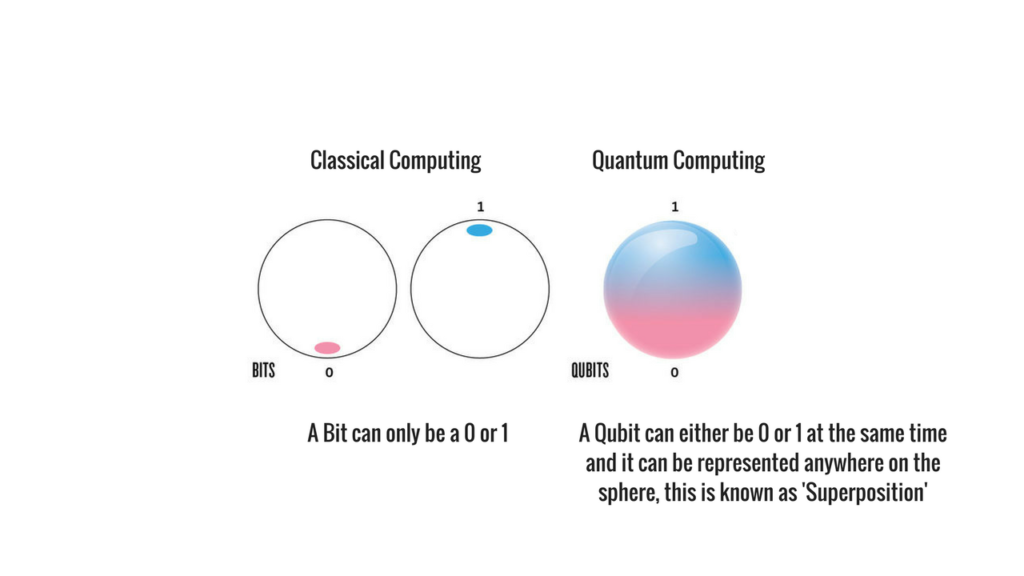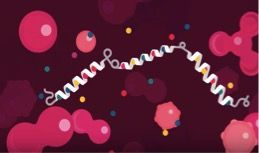Over the past two decades, there has been a tremendous rise in the evolution of technology, thereby growing businesses to greater levels. From banking to finance, to e-commerce and entertainment, technology has done great wonders.

One out of a thousand advancements is the advancement in bit computing, called as the Quantum Computing. Computing power of any computer majorly depends on its processor, that is, the number of integrated circuits. However, the basic computing depends only on 2 bits, either a 0 or a 1. No matter how many chips or precisely, how many transistors we integrate, this processor can have only one state out of the two (0 or 1) at a time. Another aspect of classical computing is that the memory is made of bits, and one bit can hold only one value out of 0 and 1. This reduces the overall capacity of the system to hold larger data, such as in trillions. A quantum computer however, can take both bits – 0 and 1 simultaneously, and hence, gives an edge over classical computers! The question is, HOW???IBM’s Quantum Computer – outranked all other quantum computers by attaining 56-Qubit quantum supremacy which simulated with only 4.5 terabytes of memory.

Quantum computers use quantum bits popularly known as Qubits. These qubits may consist of both 0 and 1. Now, when we say it consists of both 0 and 1, we mean that there is a state such that it possesses the characteristics of both state 0 and state 1. This has been achieved by the concepts of SUPERPOSITION and ENTANGLEMENT.

The superposition principle states that at a time, the qubit can exists in one or more of the possible states, the possible states being 2n, where n is the number of qubits. For instance, if there is a 2-qubit quantum computer, the possible states in which it can exists would be 22 = 4 [00, 01, 10, 11], simultaneously in 0 and 1!

Entanglement proposes to the concept of correlation of qubits. Imagine 2 qubits, each in the state |0> + |1> (a superposition of 0 and 1). We can entangle the 2 qubits such that the measurement of one qubit is always correlated to the measurement of other qubit.Let us try to understand difference between a bit and a qubit in an easier way. Consider a sphere. A bit can be either in state 0 or 1. This means it can be on either of the two poles of the sphere.However, a qubit can be in either of the two states or even in superposition of both the states. So it can lie anywhere on the sphere. Hence qubits are able to make the most out of the same space that a bit can make in a classical computer. A qubit can store 2n states in just n bits, while a classical computer would only be able to store n states in n bits. This is why storage of large information is possible in a quantum computer. So where do you think these large calculations come from?

Applications of Quantum Computers:

There are a number of fields where quantum theory excels classical theory. Some of the most critical out of them are:

1. Biomedical Simulations:

Quantum Simulation of molecules such as proteins and other atoms and ions can lead to development of better medicines and treatments to treat chronic illness.2. Machine Learning and Big Data:

Artificial Intelligence requires large datasets to be able to extract information. We already have a huge amount of data today (around 7-8 Zettabytes already existed until 2015!). This data can be well analyzed by quantum computer, rather than using distributed computing concept.

3. Optimization:

Let us say there is a salesman who needs to travel to cities for selling his products. He wants to find an optimal route he can take to cover all cities in minimal time. With few cities, it can be solved on a classical computer. However, with just 300 cities to cover, he would have more routes than total atoms present in the Universe!! Classical computers cannot handle such a large number. Hence quantum computing proves far more efficient as the solution for optimization.

Challenges:

300 perfectly entangled qubits in superposition could map all the information in the universe starting from the Big Bang!! Then what are we waiting for???

With every revolutionizing technology, comes heating challenges.

When we talk about superposition of qubits, this superposition stays within the system until we disturb the system to measure the state. These superpositions cannot stay for long and hence, by the time we are ready to do the calculations based on these superpositions, other factors such as heat, pressure, temperature, magnetic interference, etc. destroys the superposition, bringing its operation to same as classical computing. This is called decoherence.

Decoherence is the biggest challenge that quantum world is dealing with. We need to preserve the results until we can record them. This challenge is being looked upon by various error correction theories.

Conclusion:

Talking such a length on quantum computing might make you think this would replace classical computers in near future. But this is not the case.

Classical computers can never be replaced completely by quantum computers. Quantum computers have been designed to solve problems that classical computers cannot. But there are several factors which give an edge to classical computers over quantum computers.

• Cost of designing quantum computers is very high.
• They require very intrigued designing, which can only be done by people having extreme knowledge of the technology and subject.
• The end users are more familiar with classical computers.
• Quantum systems are very less in numbers.

Classical computers are never going out of fashion. But quantum computers still have a long way to go!